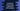# JavaScript Array isArray method explanation with examples## JavaScript Array isArray method explanation with examples:

The isArray() is a useful method to check if something is an array or not. For example, if you are getting data from the server and you want to verify if the data is an array of objects. You can simply use this method to check that.

It returns a boolean value to define if a value is an array or not. In this post, we will learn how this method works with different examples.

### Definition of isArray:

The syntax of isArray method is:

``Array.isArray(v)``

Where v is the value to check. This is the only parameter it takes.

#### Return value of isArray:

isArray method returns a boolean value. It returns true if the value is an Array, else false.

### Example of isArray:

Let’s try isArray with an example:

``````let arr = [1, 2, 3, 4];
let str = "hello";
let num = 100;

console.log(Array.isArray(arr));
console.log(Array.isArray(str));
console.log(Array.isArray(num));``````

For this example, we are trying isArray with three different variables. arr is an array, str is a string and num is a number.

If you run this program, it will print:

``````true
false
false``````

As you can see here, the first console.log prints true as isArray returned true for the array and the other log statements prints false.

We can use the return value with a if-else block before operating on any array. For example:

``````const printArray = (arr) => {
if (Array.isArray(arr)) {
for (i in arr) {
console.log(i);
}
} else {
console.log("Not an array !");
}
};

printArray([1, 2, 3, 4]);
printArray("hello");
printArray(123);``````

In this example, we are calling printArray method to print an array. It uses Array.isArray to verify if the parameter is an array or not. If it is not an array, it prints one message. Otherwise, it prints the content of the array.

If you run this program, it will print the content of the array that we are calling in the first printArray call. For the second and third calls, it will print that it is not an array.

``````0
1
2
3
Not an array !
Not an array !``````

Before processing on an array, we can use Array.isArray to check if the data is an array or not.

### Example of isArray with object array:

Array.isArray method works similarly with an object array as well. Let me show you an example:

``````const isArray = (arr) => {
return Array.isArray(arr);
};

console.log(
isArray([
{ name: "Alex", age: 20 },
{ name: "Bob", age: 21 },
{ name: "Charlie", age: 19 },
])
);

console.log(isArray({ name: "Alex" }));``````

Here,

• isArray method returns the result of Array.isArray, i.e. a boolean value, true or false.
• We are printing the logs for one array of objects and for one single object.

If you run this program, it will print the below output:

``````true
false``````

As you can see here, it prints true for the array and it prints false for the single object. So, you can use this method to check even for an array of objects.